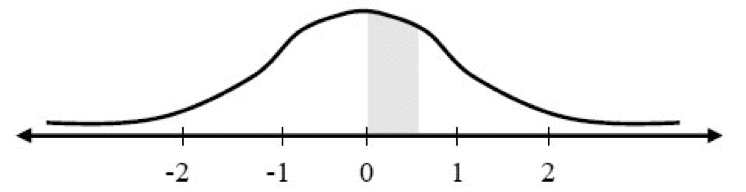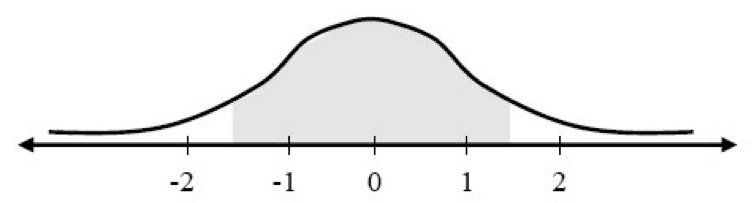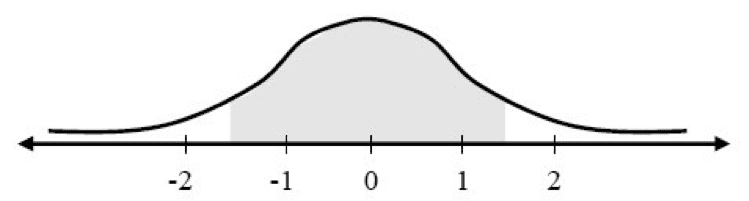# Events with a Starting Point and an End Point

Question: What is the probability of obtaining values between 0 and 0.5 (i.e., the area shaded in gray)?

DiagramExplanation: In order to find the area shaded in gray, the following subtractions should be performed:

 The area under the curve to the left of 0.5 = 0.6915 The area under the curve to the left of 0 = 0.5000 The result 0.1915

Question: What is the probability of obtaining values between –1.5 and 1.5?

DiagramExplanation: The area under the curve to the left of 1.5 is 0.9332, and the area under the curve to the left of -1.5 is 0.0688. Subtracting the second number from the first will provide the area shaded in the diagram, which is the desired probability: 0.9332 – 0.0668 = 0.8664.

Question: What is the probability of obtaining values greater than 1.5 or less than –1.5?

Diagram